## 一、问题描述

CIFAR-10数据集有60000张图片，每张图片均为分辨率为32*32的彩色图片（分为RGB3个信道）。CIFAR-10的分类任务是将每张图片分成青蛙、卡车、飞机等10个类别中的一个类别。本文主要使用基于卷积神经网络的方法（CNN）来设计模型，完成分类任务。

## 二、搭建最简单版本的CNN

1. 卷积层1：卷积核大小3*3，卷积核移动步长1，卷积核个数64，池化大小2*2，池化步长2，池化类型为最大池化，激活函数ReLU。
2. 卷积层2：卷积核大小3*3，卷积核移动步长1，卷积核个数128，池化大小2*2，池化步长2，池化类型为最大池化，激活函数ReLU。
3. 卷积层3：卷积核大小3*3，卷积核移动步长1，卷积核个数256，池化大小2*2，池化步长2，池化类型为最大池化，激活函数ReLU。
4. 全连接层：隐藏层单元数1024，激活函数ReLU。
5. 分类层：隐藏层单元数10，激活函数softmax。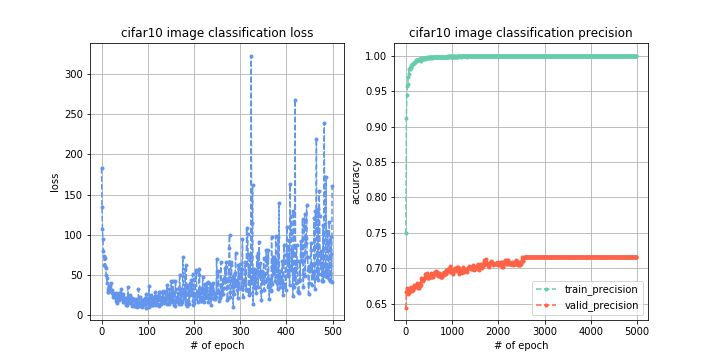## 三、数据增强有很大的作用

1. 图像切割：生成比图像尺寸小一些的矩形框，对图像进行随机的切割，最终以矩形框内的图像作为训练数据。
2. 图像翻转：对图像进行左右翻转。
3. 图像白化：对图像进行白化操作，即将图像本身归一化成Gaussian(0,1)分布。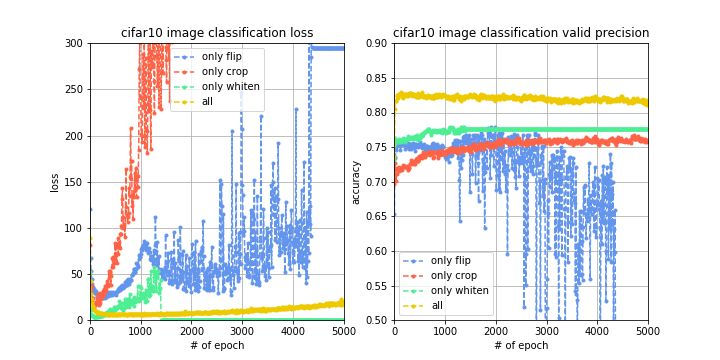## 四、从模型入手，使用一些改进方法

1. 权重衰减（weight decay）：对于目标函数加入正则化项，限制权重参数的个数，这是一种防止过拟合的方法，这个方法其实就是机器学习中的l2正则化方法，只不过在神经网络中旧瓶装新酒改名为weight decay 。
2. dropout：在每次训练的时候，让某些的特征检测器停过工作，即让神经元以一定的概率不被激活，这样可以防止过拟合，提高泛化能力 。
3. 批正则化（batch normalization）：batch normalization对神经网络的每一层的输入数据都进行正则化处理，这样有利于让数据的分布更加均匀，不会出现所有数据都会导致神经元的激活，或者所有数据都不会导致神经元的激活，这是一种数据标准化方法，能够提升模型的拟合能力 。
4. LRN：LRN层模仿生物神经系统的侧抑制机制，对局部神经元的活动创建竞争机制，使得响应比较大的值相对更大，提高模型泛化能力。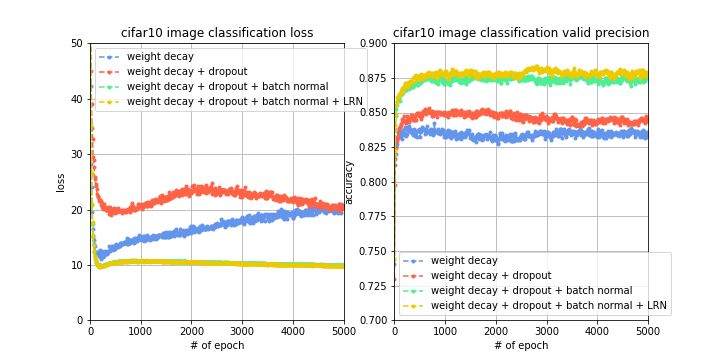## 五、变化的学习率，进一步提升模型性能

1. 首先使用较大的学习率进行训练，观察目标函数值和验证集准确率的收敛曲线。
2. 如果目标函数值下降速度和验证集准确率上升速度出现减缓时，减小学习率。
3. 循环步骤2，直到减小学习率也不会影响目标函数下降或验证集准确率上升为止。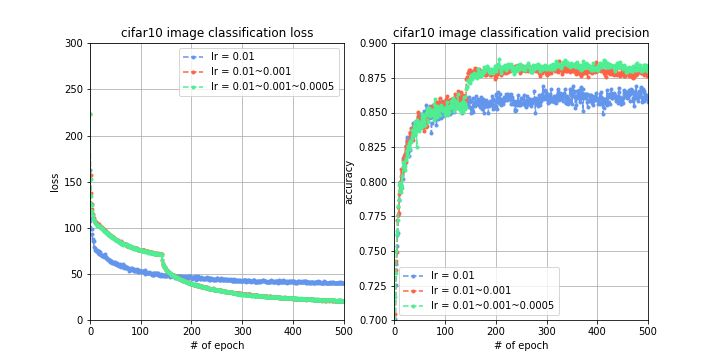## 六、加深网络层数，会发生什么事情？

1. 卷积层1：卷积核大小3*3，卷积核移动步长1，卷积核个数16，激活函数ReLU，使用batch_normal和weight_decay，接下来的n层，卷积核大小3*3，卷积核移动步长1，卷积核个数16，激活函数ReLU，使用batch_normal和weight_decay。
2. 卷积层2：卷积核大小3*3，卷积核移动步长2，卷积核个数32，激活函数ReLU，使用batch_normal和weight_decay，接下来的n层，卷积核大小3*3，卷积核移动步长1，卷积核个数32，激活函数ReLU，使用batch_normal和weight_decay。
3. 卷积层3：卷积核大小3*3，卷积核移动步长2，卷积核个数64，激活函数ReLU，使用batch_normal和weight_decay，接下来的n层，卷积核大小3*3，卷积核移动步长1，卷积核个数64，激活函数ReLU，使用batch_normal和weight_decay。
4. 池化层：使用全局池化，对64个隐藏单元分别进行全局池化。
5. 全连接层：隐藏层单元数10，激活函数softmax，使用batch_normal和weight_decay。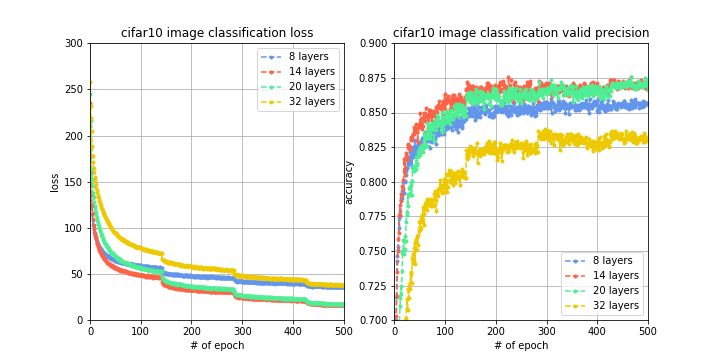## 七、终极武器，残差网络

2015年，Microsoft用残差网络  拿下了当年的ImageNet，这个残差网络就很好地解决了梯度衰减的问题，使得深度神经网络能够正常work。由于网络层数加深，误差反传的过程中会使梯度不断地衰减，而通过跨层的直连边，可以使误差在反传的过程中减少衰减，使得深层次的网络可以成功训练，具体的过程可以参见其论文。

### 同的改进策略对分类准确率的影响，如何一步步得提高准确率。

``import numpy as np# 序列化和反序列化import picklefrom sklearn.preprocessing import OneHotEncoderimport warningswarnings.filterwarnings('ignore')import tensorflow as tf  #数据加载 def unpickle(file):    import pickle    with open(file, 'rb') as fo:        dict = pickle.load(fo, encoding='ISO-8859-1')    return dictlabels = []X_train = []for i in range(1,6):    data = unpickle('./cifar-10-batches-py/data_batch_%d'%(i))    labels.append(data['labels'])    X_train.append(data['data'])# 将list类型转换为ndarrayX_train = np.array(X_train) X_train = np.transpose(X_train.reshape(-1,3,32,32),[0,2,3,1]).reshape(-1,3072) y_train = np.array(labels).reshape(-1) # reshapeX_train = X_train.reshape(-1,3072) # 目标值概率one_hot = OneHotEncoder()y_train =one_hot.fit_transform(y_train.reshape(-1,1)).toarray() # 测试数据加载test = unpickle('./cifar-10-batches-py/test_batch')X_test = test['data']X_test = np.transpose(X_test.reshape(-1,3,32,32),[0,2,3,1]).reshape(-1,3072)y_test = one_hot.transform(np.array(test['labels']).reshape(-1,1)).toarray()  # 从总数据中获取一批数据index = 0def next_batch(X,y):    global index    batch_X = X[index*128:(index+1)*128]    batch_y = y[index*128:(index+1)*128]    index+=1    if index == 390:        index = 0    return batch_X,batch_y    # 构建神经网络  X = tf.placeholder(dtype=tf.float32,shape = [None,3072])y = tf.placeholder(dtype=tf.float32,shape = [None,10])kp = tf.placeholder(dtype=tf.float32) ### ！！！变量def gen_v(shape,std = 5e-2):    return tf.Variable(tf.truncated_normal(shape = shape,stddev=std)) def conv(input_,filter_,b):    conv = tf.nn.conv2d(input_,filter_,strides=[1,1,1,1],padding='SAME') + b    conv = tf.layers.batch_normalization(conv,training=True)    conv = tf.nn.relu(conv)    return tf.nn.max_pool(conv,[1,3,3,1],[1,2,2,1],'SAME') def net_work(X,kp):#     形状改变，4维    input_ = tf.reshape(X,shape = [-1,32,32,3])#     第一层    filter1 = gen_v(shape = [3,3,3,64])    b1 = gen_v(shape = )    pool1 = conv(input_,filter1,b1)    #     第二层    filter2 = gen_v([3,3,64,128])    b2 = gen_v(shape = )    pool2 = conv(pool1,filter2,b2)    #     第三层    filter3 = gen_v([3,3,128,256])    b3 = gen_v()    pool3 = conv(pool2,filter3,b3)    #     第一层全连接层    dense = tf.reshape(pool3,shape = [-1,4*4*256])    fc1_w = gen_v(shape = [4*4*256,1024])    fc1_b = gen_v()    bn_fc_1 = tf.layers.batch_normalization(tf.matmul(dense,fc1_w) + fc1_b,training=True)    relu_fc_1 = tf.nn.relu(bn_fc_1)#     fc1.shape = [-1,1024]        #     dropout    dp = tf.nn.dropout(relu_fc_1,keep_prob=kp)    #     fc2 输出层    out_w = gen_v(shape = [1024,10])    out_b = gen_v(shape = )    out = tf.matmul(dp,out_w) + out_b    return out     # 损失函数准确率&最优化 out = net_work(X,kp)loss = tf.reduce_mean(tf.nn.softmax_cross_entropy_with_logits_v2(labels=y,logits=out))# 准确率y_ = tf.nn.softmax(out)# equal 相当于 == equal = tf.equal(tf.argmax(y,axis = -1),tf.argmax(y_,axis = 1))accuracy = tf.reduce_mean(tf.cast(equal,tf.float32))  opt = tf.train.AdamOptimizer(0.01).minimize(loss)opt  # 开启训练 saver = tf.train.Saver() # config = tf.ConfigProto(allow_soft_placement=True)# config.gpu_options.allocator_type = 'BFC'# config.gpu_options.per_process_gpu_memory_fraction = 0.80 epoches = 100with tf.Session() as sess:    sess.run(tf.global_variables_initializer())    for i in range(epoches):        batch_X,batch_y = next_batch(X_train,y_train)        opt_,loss_ ,score_train= sess.run([opt,loss,accuracy],feed_dict = {X:batch_X,y:batch_y,kp:0.5})        print('iter count:%d。mini_batch loss:%0.4f。训练数据上的准确率：%0.4f。测试数据上准确率：...'%              (i+1,loss_,score_train ))        if score_train > 0.6:            saver.save(sess,'./model/estimator',i+1)    saver.save(sess,'./model/estimator',i+1)     for i in range(77):        X_test1,y_test1=  next_batch(X_test,y_test)         score_test = sess.run([accuracy],feed_dict = {X:X_test1,y:y_test1,kp:1.0})        print('测试数据上的准确率：',score_test,y_,y)  epoches = 1100with tf.Session() as sess:    saver.restore(sess,'./model/estimator-100')    for i in range(100,epoches):        batch_X,batch_y = next_batch(X_train,y_train)        opt_,loss_ ,score_train= sess.run([opt,loss,accuracy],feed_dict = {X:batch_X,y:batch_y,kp:0.5})        print('iter count:%d。mini_batch loss:%0.4f。训练数据上的准确率：%0.4f。测试数据上准确率：%0.4f'%              (i+1,loss_,score_train,score_test))        if score_train > 0.6:            saver.save(sess,'./model/estimator',i+1)    saver.save(sess,'./model/estimator',i+1)    if (i%100 == 0) and (i != 100):        score_test = sess.run(accuracy,feed_dict = {X:X_test,y:y_test,kp:1.0})        print('----------------测试数据上的准确率：---------------',score_test)``